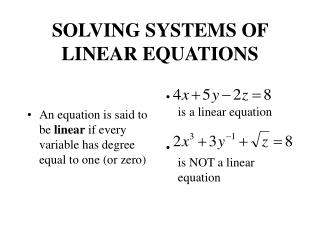DownloadDownload PresentationSOLVING SYSTEMS OF LINEAR EQUATIONS

# SOLVING SYSTEMS OF LINEAR EQUATIONS

Download Presentation## SOLVING SYSTEMS OF LINEAR EQUATIONS

- - - - - - - - - - - - - - - - - - - - - - - - - - - E N D - - - - - - - - - - - - - - - - - - - - - - - - - - -
##### Presentation Transcript

1. An equation is said to be linear if every variable has degree equal to one (or zero) is a linear equation is NOT a linear equation SOLVING SYSTEMS OF LINEAR EQUATIONS

2. Review these familiar techniques for solving 2 equations in 2 variables. The same techniques will be extended to accommodate larger systems. Times 3 Add Substitute to solve: y=1

3. L1 is replaced by 3L1 L1 is replaced by L1 + L2 L1 is replaced by (1/7)L1 L1 represents line oneL2 represents line two

4. These systems are said to be EQUIVALENT because they have the SAME SOLUTION.

5. PERFORM ANY OF THESE OPERATIONS ON A SYSTEM OF LINEAR EQUATIONS TO PRODUCE AN EQUIVALENT SYSTEM: • INTERCHANGE two equations (or lines) • REPLACE Ln with k Ln , k is NOT ZERO • REPLACE Ln with Ln + cLm • note: Ln is always part of what replaces it.

6. L1 L3 L2 L1 L2 L3 is equivalent to L1 L2 L3 L1 4L2 L3 is equivalent to L1 L2 L3 L1 L2 L3 + 2L1 is equivalent to EXAMPLES:

7. example

8. - + = x 2 y 4 z 0 - + - = x 3 y 4 z 3 = z 1 - + = x 2 y 4 z 0 = y 3 = z 1 = x +4z 6 = y 3 = z 1 Replace L1 with (1/2) L1 Replace L3 with L3 + L2 Replace L2 with L2 + L1 Replace L1 with L1 + 2 L2

9. Replace L1 with L1 + - 4 L3

10. is EQUIVALENT to = x 2 = y 3 = z 1

11. one more example

12. To solve the following system, we look for an equivalent system whose solution is more obvious. In the process, we manipulate only the numerical coefficients, and it is not necessary to rewrite variable symbols and equal signs:

13. This rectangular arrangement of numbers is called a MATRIX

14. 2 Replace L1 with L1 + 2 L2

15. 1 Replace L3 with L3 + L2

16. Interchange L2 and L3

17. Replace L1 with L1 + -1 L2 Replace L2 with -1 L2 Replace L3 with -1 L3

18. The original matrix represents a system that is equivalent to this final matrix whose solution is obvious

19. The original matrix represents a system that is equivalent to this final matrix whose solution is obvious

20. The diagonal of ones The zeros Note the format of the matrix that yields this obvious solution: Whenever possible, aim for this format.